### 1，数据归一化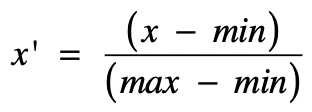• x 是当前要变换的原始值。
• min 是当前特征中的最小值。
• max 是当前特征中的最大值。
• x' 是变换完之后的新值。

#### 1.1，归一化处理

• Max(特征1) = 69，Min(特征1) = 5
• Max(特征2) = 796，Min(特征2) = 378
• Max(特征3) = 135，Min(特征3) = 69

• 对于第一个数字 5 做变换：(5 - 5) / (69 - 5) = 0
• 对于第二个数字 465 做变换：(465 - 378) / (796 - 378) = 0.21
• 对于第三个数字 135 做变换：(135 - 69) / (135 - 69) = 1

#### 1.2，使用 MinMaxScaler 类

sklearn 库的 preprocessing 模块中的 MinMaxScaler 类就是用来做归一化处理的。

>>> from sklearn.preprocessing import MinMaxScaler


>>> data = [[5, 465, 135], [23, 378, 69], [69, 796, 83]]
>>> scaler = MinMaxScaler() # 默认将数据拟合到 [0, 1] 范围内


>>> scaler.fit(data)


>>> scaler.data_max_         # 特征最大值
array([ 69., 796., 135.])
>>> scaler.data_min_         # 特征最小值
array([  5., 378.,  69.])


>>> scaler.transform(data)
array([[0.        , 0.20813397, 1.        ],
[0.28125   , 0.        , 0.        ],
[1.        , 1.        , 0.21212121]])


### 2，数据标准化

z-score 标准化是基于正态分布的，该方法假设数据呈现标准正态分布

#### 2.1，什么是正态分布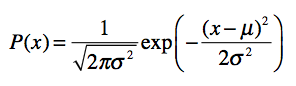• 正态分布以经过均值 u 的垂线为轴，左右对称展开，中间点最高，然后逐渐向两侧下降。
• 分布曲线和 X 轴组成的面积为 1，表示所有事件出现的概率总和为 1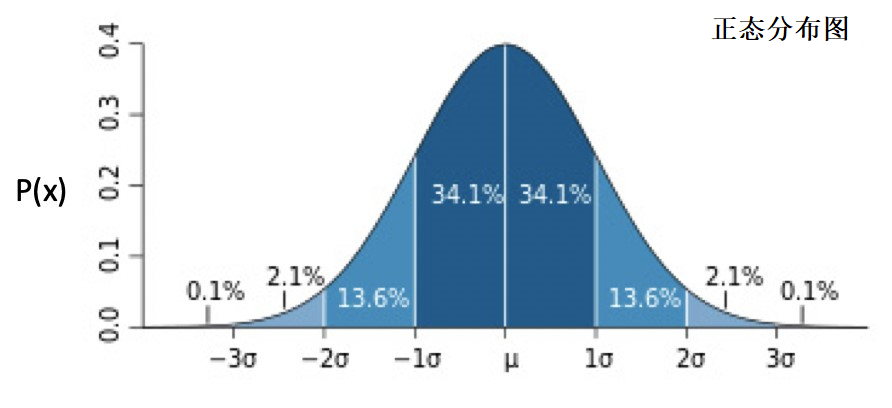μ0σ1时，正态分布为标准正态分布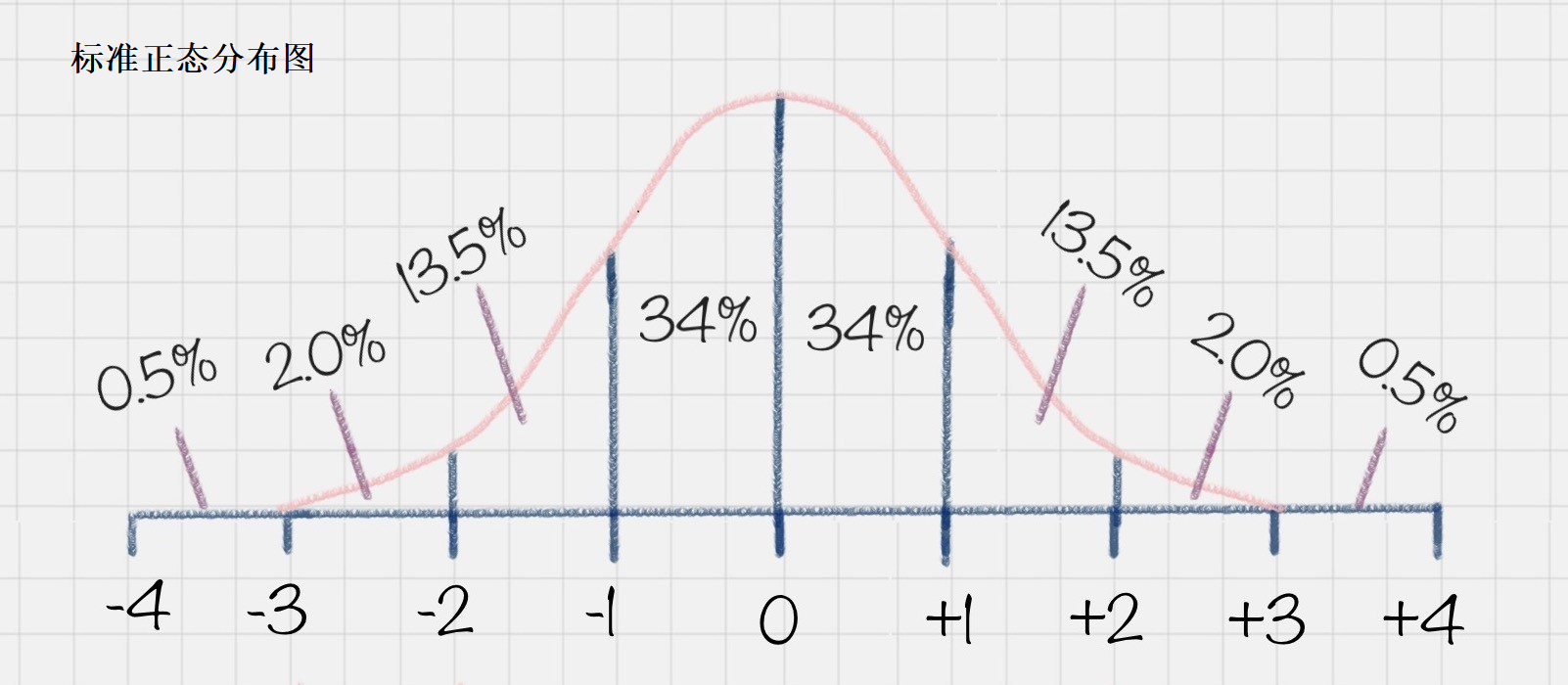#### 2.2，z-score 标准化

z-score 标准化利用正态分布的特点，计算一个给定分数距离平均数有多少个标准差。它的转换公式如下：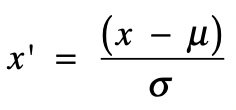#### 2.3，使用 StandardScaler 类

sklearn 库的 preprocessing 模块中的 StandardScaler 类就是用来做z-score 标准化处理的。

>>> from sklearn.preprocessing import StandardScaler


>>> data = [
[5, 465, 135],
[23, 378, 69],
[69, 796, 83]
]
>>> scaler = StandardScaler()


>>> scaler.fit(data)


>>> scaler.mean_   # 均值
array([ 32.33333333, 546.33333333,  95.66666667])
>>> scaler.scale_  # 标准差
array([ 26.94851058, 180.078378  ,  28.39405259])


>>> scaler.transform(data)
array([[-1.01427993, -0.45165519,  1.38526662],
[-0.34633949, -0.93477815, -0.93916381],
[ 1.36061941,  1.38643334, -0.44610281]])


### 3，总结

• 归一化：使用特征值中的最大最小值，把原始值转换为 0 到 1 之间的值。
• 优点：是简单易行，好理解。
• 缺点：是容易受最大最小值的干扰。
• 介绍了 MinMaxScaler 类的使用。
• 标准化：介绍了 z-score 标准化，原始数据经过转换后，符合标准正态分布。
• 和归一化相比，z-score 标准化不容易受到噪音数据的影响。
• 介绍了 StandardScaler 类的使用。

（本节完。）